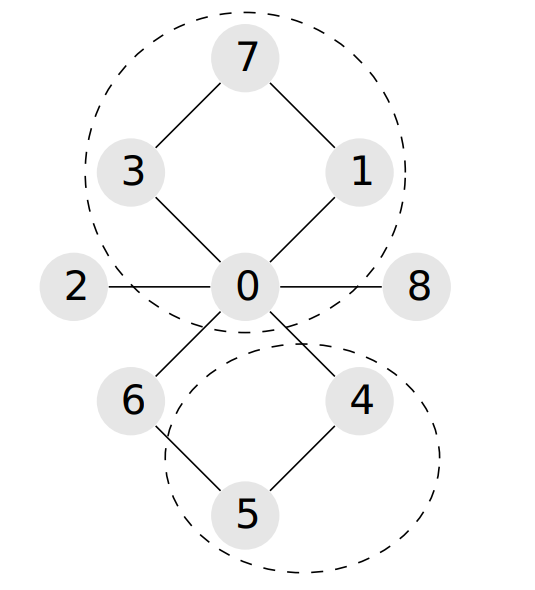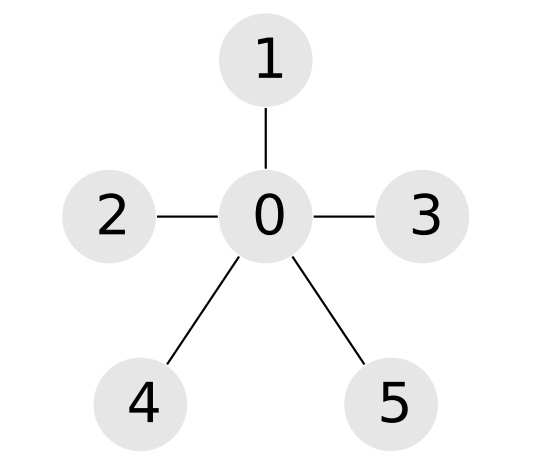TouchStone
P5961「IOI2019」景点划分
 制限 : 時間制限 : - MS   メモリ制限 : - KB審判説明 : 2s,1024m
###### 問題説明

Fatima 打算在三天之内参观完所有这些景点。她已经决定要在第一天参观 $a$ 处景点，第二天参观 $b$ 处景点，第三天参观 $c$ 处景点。因此，她要把这 $n$ 处景点划分为三个集合 $A,B$$C$，其规模分别为 $a,b$$c$。每处景点恰好属于其中一个集合，因此有 $a+b+c=n$

Fatima 想要找到这样的集合划分 $A,B$$C$，使得这三个集合中的至少两个连通的。一个景点集合 $S$ 被称为是连通的，如果能够经由这些道路在 $S$ 中的任意两处景点之间往来，且不需要经过不在 $S$ 中的景点。如果满足上述要求，则景点的一个划分 $A,B$$C$ 被称为是合法的

#### 样例输入 1

9 10
4 2 3
0 1
0 2
0 3
0 4
0 6
0 8
1 7
3 7
4 5
5 6


#### 样例输出 1

1 1 3 1 2 2 3 1 3


#### 样例说明 1#### 样例输入 2

6 5
2 2 2
0 1
0 2
0 3
0 4
0 5


#### 样例输出 2

0 0 0 0 0 0


#### 样例说明 2• $3\le n\le 10^5$；
• $2\le m\le 2\times 10^5$；
• $1\le a,b,c\le n,a+b+c=n$；
• 每一对景点之间至多有一条道路；
• 经由这些道路，可以在任意两处景点之间往来；
• 对于 $1\le i\le m$，有 $0\le p_i,q_i\le n-1$ 和 $p_i\neq q_i$

$1$ 每处景点至多可做两条道路的端点 $7$
$2$ $a=1$ $11$
$3$ $m=n-1$ $22$
$4$ $n\le 2.5\times 10^3,m\le 5\times 10^3$ $24$
$5$ 没有任何附加限制 $36$